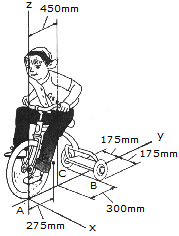# Engineering Mechanics - Equilibrium of a Rigid Body - Discussion

### Discussion :: Equilibrium of a Rigid Body - General Questions (Q.No.1)

1.The girl has a mass of 17kg and mass center at Gg, and the tricycle has a mass of 10kg and mass center at Gt. Determine the normal reactions at each wheel for equilibrium.

 [A]. NA = 14.77 N, NB = NC = 6.12 N [B]. NA = 128.8 N, NB = NC = 68.0 N [C]. NA = 144.9 N, NB = NC = 60.0 N [D]. NA = 13.15 N, NB = NC = 6.93 N

Explanation:

No answer description available for this question.

 Sam said: (Sep 22, 2012) Force at Gg = 17g N where g=9.81 Force at Gt = 10g N Distance Gg -> A = 450 Distance Gg -> CD = 300 Force A = 300/750 * 17g = 66.6 N Force CD = 450/750 * 17g = 100N Distance Gt -> A = 275 Distance Gt -> CD = 425 Force A = 425/700 * 10g = 59.5N Force CD = 275/700 * 10g = 38.5N Total force A = 59.5+66.4 = 126 N (which is close approx.) Total force CD = 100+38.5 = 138.5N Force at each wheel = 138.5/2 = 69N (approx.) B is the closest answer

 Sreerag said: (Sep 18, 2014) Please answer the question that 3 cylinders are placed in a rectangular channel or shown in the figure. Determine the reaction between the cylinder 1 and vertical wall.